﻿ On a Certain Identity Involving the Gamma FunctionPublications are Open
Access in this journal
Article Versions
Export Article
• Normal Style
• MLA Style
• APA Style
• Chicago Style
Research Article
Open Access Peer-reviewed

### On a Certain Identity Involving the Gamma Function

Theophilus Agama
American Journal of Mathematical Analysis. 2021, 9(1), 1-5. DOI: 10.12691/ajma-9-1-1
Received January 08, 2021; Revised February 09, 2021; Accepted February 23, 2021

### Abstract

The goal of this paper is to prove the identity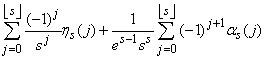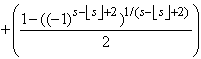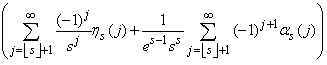whereand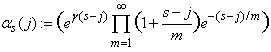whereis the Gamma function defined by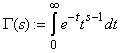and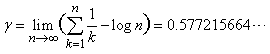is the Euler-Mascheroni constant.

### 1. Introduction

The Euler-Gamma function is defined by,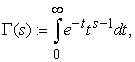valid in the entire complex plane, except at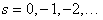where it has simple poles 1. It can also be seen as a generalization of the factorial on the positive integers to the rationals. Indeed the Gamma function (See 1, 2) satisfies the functional equation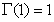andso that in the case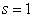and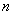is a positive integer, then we have the expression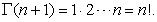The Gamma function still remains valid for arguments in the range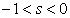by the equation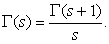It also has the canonical product representation (See 3)valid for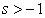. The gamma function also has very key properties, most notably the duplication and the complementary property (reflexive formula), which are given respectively as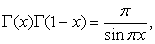and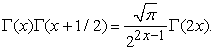For many more of these properties, the reader is encouraged to see 1. The Gamma function is also inextricably linked to some very interesting functions. Consider the digamma function 1, the logarithmic derivative of the Gamma function defined by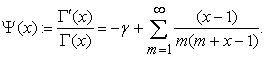The Gamma funtion has spawn a great deal of research and out of which has led to the discovery of many beautiful identities and inequalities. More recently the gamma function has been studied by Alzer and many other authors. For more results on the gamma function, see 2, 3. In this paper, however, we prove a certain identity related to the Gamma function.

### 2. Main Theorem

Theorem 2.1. For any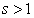, we havewhere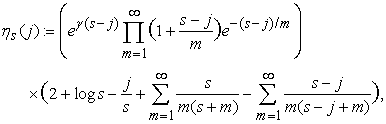andwhere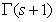is the Gamma function defined by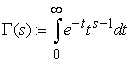andis the Euler-Mascheroni constant.

Proof. Letbe a real-valued function, contineously differentiable on the intervalandfor all. Then we set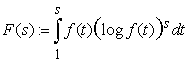for. In the simplest case, we choose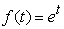, since it satisfies the hypothesis. Thus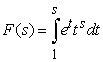. By application of integration by parts, we find that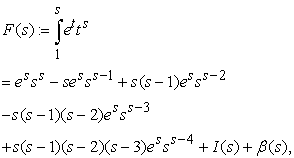whereand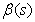are convergent. More precisely, we can writein a closed form as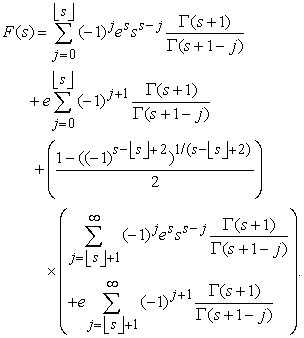Now, since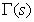is analytic in the half plane, it follows by the convergence of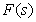that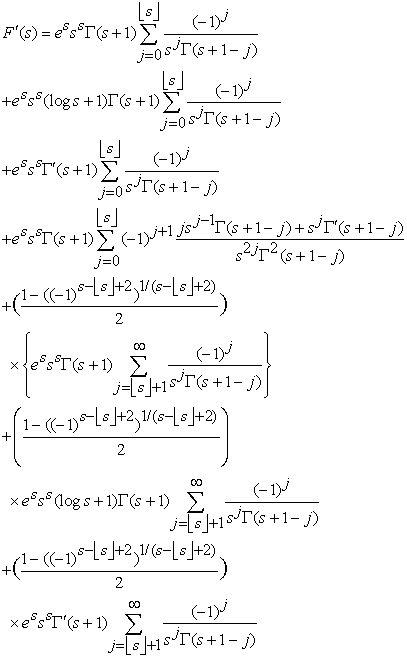On the other hand. Arranging terms and comparing both results we find that(2.1)

Using the following identities involving the Gamma function 1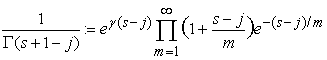(2.2)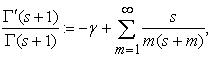(2.3)

the remaining task is to arrange the terms and apply these identities and identify the functionand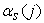. We leave the remaining task to the reader to verify.

Remark 2.2. Now we examine some immediate conequences of the above result, in the following sequel.

Corollary 1. The identity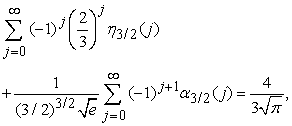where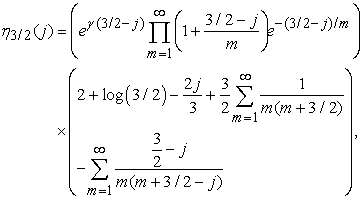andremains valid.

Proof. Let us set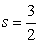in Theorem 2.1. Then it follows thatwhere we have used the relation1. The proof is completed by computingandgiven in Theorem 2.1.

Corollary 2. The identity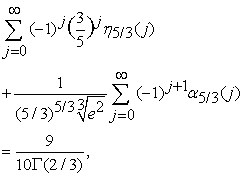is valid, whereandProof. The result follows by setting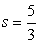in Theorem 2.1, and computing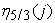and.

Corollary 3. For any integer, the inequality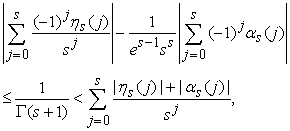whereand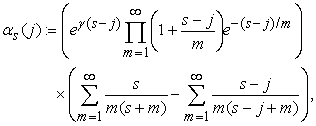is valid.

Proof. Ifis an integer, then Theorem 2.1 reduces to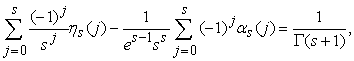and the result follows immediately by applying the triangle inequality.

### 3. Final Remarks

In this paper we proved an identity related the reciprocal of the gamma function. Consequently, we obtained the following identitieswhereand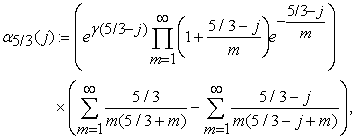and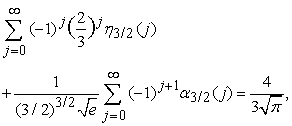withand### Conict of Interest

The author declares that he has no conict of interest in relation to this article.

### Ethical Approval

This article does not contain any studies with human participants or animals per-formed by the author.

### References

  Sebah, Pascal and Gourdon, Xavier, Introduction to the gamma function, American Journal of Scientific Research, 2002. In article  Batir, Necdet, Bounds for the gamma function, arXiv preprint arXiv:1705.06167, 2015. In article  Nantomah, Kwara and Prempeh, Edward and Twum, S. Boakye, Some inequalities for the q-Extension of the Gamma Function, arXiv preprint arXiv:1510.03459, 2015. In article

Published with license by Science and Education Publishing, Copyright © 2021 Theophilus AgamaThis work is licensed under a Creative Commons Attribution 4.0 International License. To view a copy of this license, visit http://creativecommons.org/licenses/by/4.0/